##### Circuit Analysis For DummiesUsing the Laplace transform as part of your circuit analysis provides you with a prediction of circuit response. Analyze the poles of the Laplace transform to get a general idea of output behavior. Real poles, for instance, indicate exponential output behavior.

Follow these basic steps to analyze a circuit using Laplace techniques:

1. Develop the differential equation in the time-domain using Kirchhoff’s laws and element equations.

2. Apply the Laplace transformation of the differential equation to put the equation in the s-domain.

3. Algebraically solve for the solution, or response transform.

4. Apply the inverse Laplace transformation to produce the solution to the original differential equation described in the time-domain.

To get comfortable with this process, you simply need to practice applying it to different types of circuits such as an RC (resistor-capacitor) circuit, an RL (resistor-inductor) circuit, and an RLC (resistor-inductor-capacitor) circuit.

Here you can see an RLC circuit in which the switch has been open for a long time. The switch is closed at time t = 0.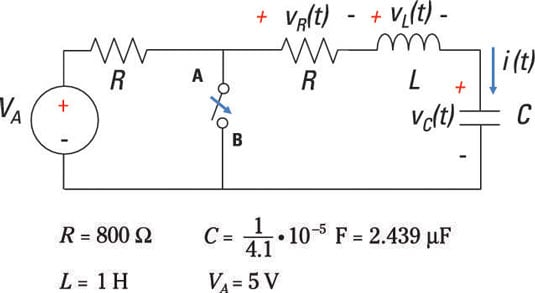In this circuit, you have the following KVL equation:

vR(t) + vL(t) + v(t) = 0

Next, formulate the element equation (or i-v characteristic) for each device. Ohm’s law describes the voltage across the resistor (noting that i(t) = iL(t) because the circuit is connected in series, where I(s) = IL(s) are the Laplace transforms):

vR(t) = i(t)R

The inductor’s element equation is given by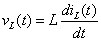And the capacitor’s element equation is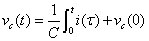Here, vC(0) = V0 is the initial condition, and it’s equal to 5 volts.

Substituting the element equations, vR(t), vC(t), and vL(t), into the KVL equation gives you the following equation (with a fancy name: the integro-differential equation):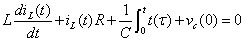The next step is to apply the Laplace transform to the preceding equation to find an I(s) that satisfies the integro-differential equation for a given set of initial conditions: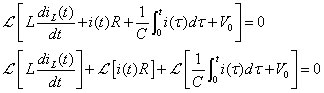The preceding equation uses the linearity property allowing you to take the Laplace transform of each term. For the first term on the left side of the equation, you use the differentiation property to get the following transform: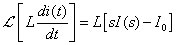This equation uses IL(s) = [i(t)], and I0 is the initial current flowing through the inductor. Because the switch is open for a long time, the initial condition I0 is equal to zero.

For the second term of the KVL equation dealing with resistor R, the Laplace transform is simply

ℒ[i(t)R] = I(s)R

For the third term in the KVL expression dealing with capacitor C, you have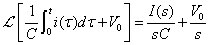The Laplace transform of the integro-differential equation becomes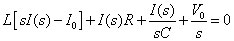Rearrange the equation and solve for I(s):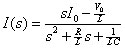To get the time-domain solution i(t), use the following table, and notice that the preceding equation has the form of a damping sinusoid.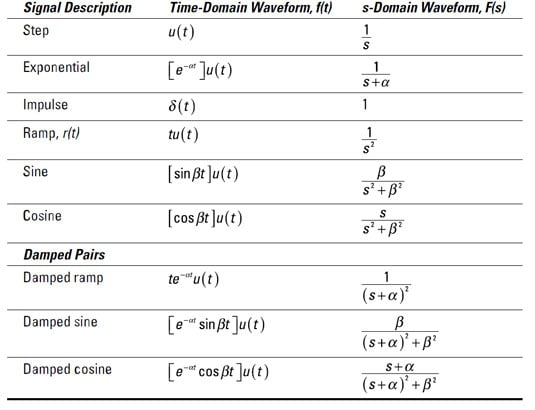Now, you plug in I0 = 0 and some numbers from this figure: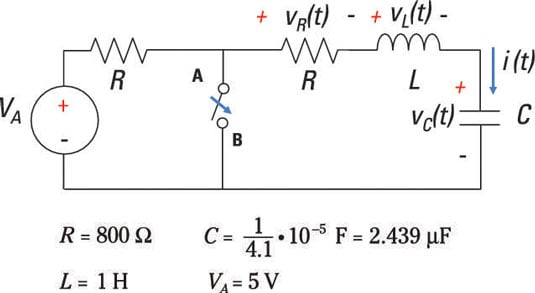Now you’ve got this equation: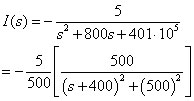You wind up with the following solution:

i(t) = [-0.01e-400t sin500t]u(t)

For this RLC circuit, you have a damping sinusoid. The oscillations will die out after a long period of time. For this example, the time constant is 1/400 and will die out after 5/400 = 1/80 seconds.